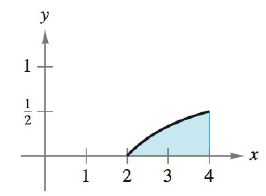Chapter 5.4, Problem 16E### Calculus: An Applied Approach (Min...

10th Edition
Ron Larson
ISBN: 9781305860919

#### Solutions

Chapter
Section### Calculus: An Applied Approach (Min...

10th Edition
Ron Larson
ISBN: 9781305860919
Textbook Problem
1 views

# Finding Area by the Fundamental Theorem In Exercises 9-16, find the area of the region. See Example 2. y = x − 2 xTo determine

To calculate: The area of the region y=x2x in the provided figure.Explanation

Given Information:

The function is y=x2x and the provided figure is:

Formula used:

The fundamental theorem of calculus states that,

If f is integrable on interval [a,b] then abf(x)dx=F(b)F(a).

The integration formulas are,

xndx=xn+1n+1+C and 1xdx=lnx+C, where C is the constant.

Calculation:

Consider the given figure.

To find the area of the region, fundamental theorem of calculus can be used.

Integrate the function from 2 to 4 as shown below,

24(x2x)dx

This can be written as,

24(

### Still sussing out bartleby?

Check out a sample textbook solution.

See a sample solution

#### The Solution to Your Study Problems

Bartleby provides explanations to thousands of textbook problems written by our experts, many with advanced degrees!

Get Started

#### Domain Find the domain of the expression. 10. 2t253t+6

Precalculus: Mathematics for Calculus (Standalone Book)

#### In Problems 39 – 44, solve each inequality and graph the solution. 42.

Mathematical Applications for the Management, Life, and Social Sciences

#### The graph of x = cos t, y = sin2 t is:

Study Guide for Stewart's Multivariable Calculus, 8th